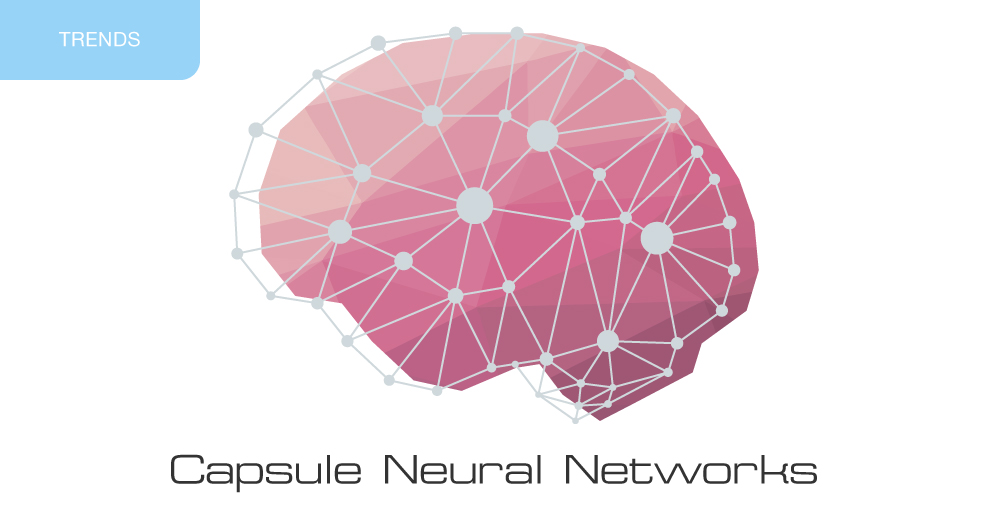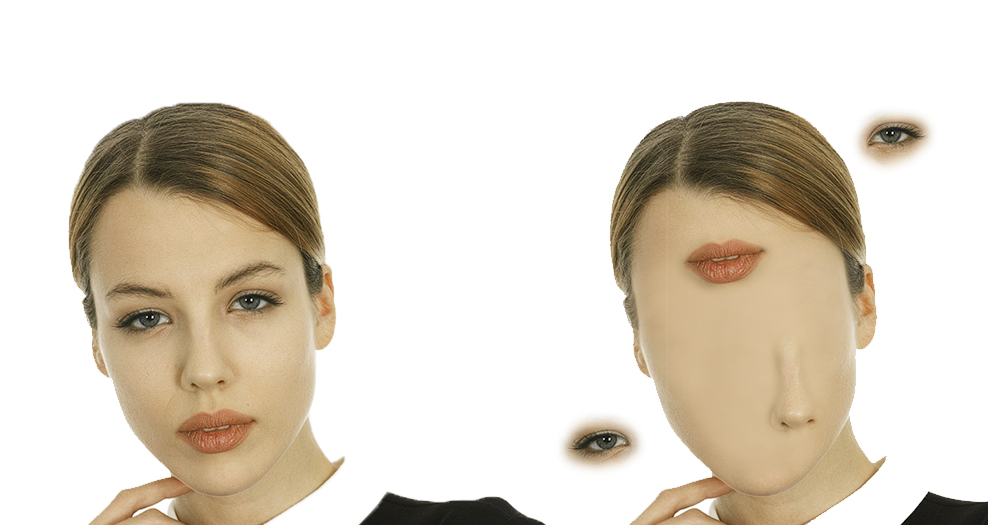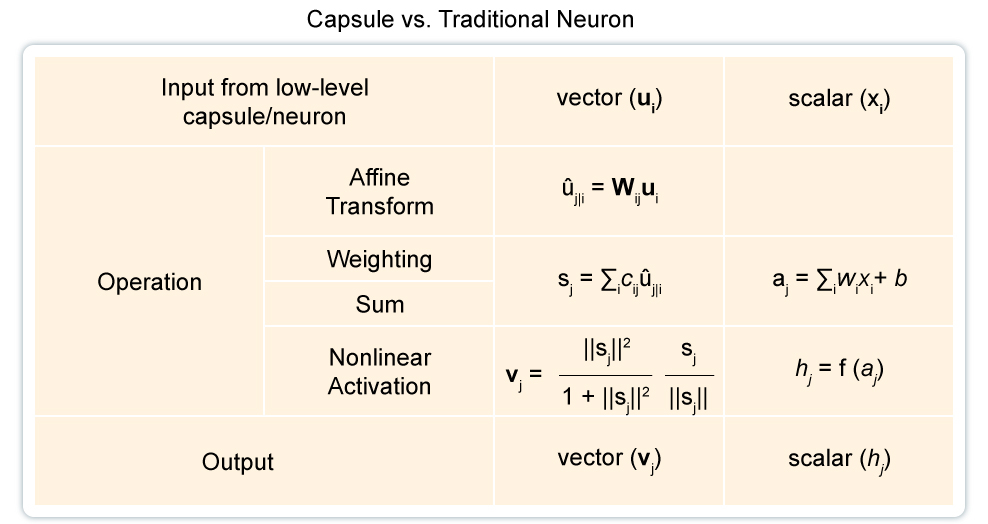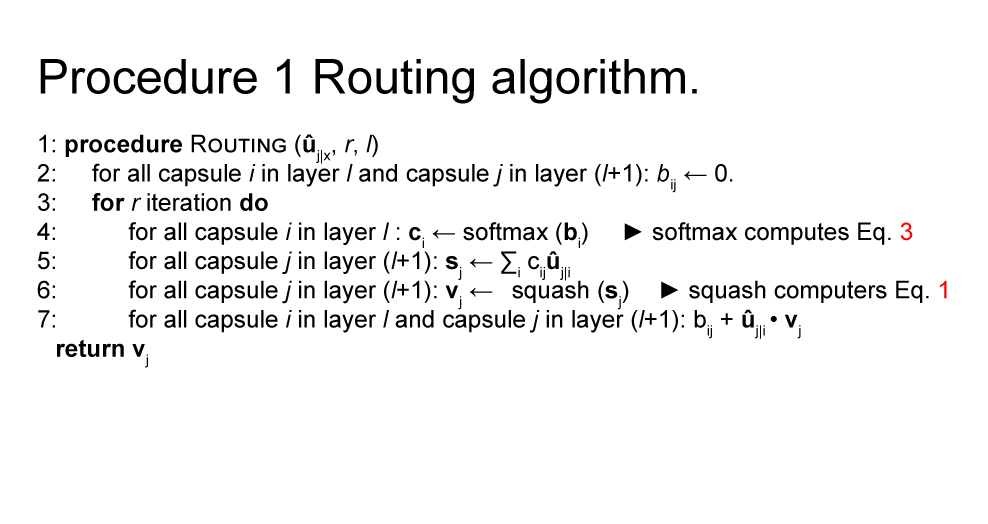# Capsule neural networksIn 2017 Geoffrey Hinton (one of the first researchers who demonstrated the use of backpropagation algorithm) published an article describing capsule neural networks and suggesting an algorithm of dynamic routing between the capsules applied for architecture teaching.

Traditional convolutional neural networks have some disadvantageous features. Inner data presentation of a convolutional neural network does not take into account space hierarchies existing between simple and complex objects. For example, if an image represents in random order a nose, eyes and lips, a convolutional neural network takes them for a face. If the image is rotated, a wrong angle of view influences negatively the quality of recognition, whereas the human brain doesn’t have any trouble recognizing rotated images.For a convolutional neural network, these two images are alike.A CNN will need thousands of examples to learn recognizing images represented from different angles.Capsule neural networks reduce an error made when recognizing an image represented from a different angle by 45%.

### The Purpose of Capsules

Capsules encapsulate the information about the state of the function they detect in the vector shape. Capsules encode the probability of detection of an object as the length of the output vector. The state of the function they have detected is encoded as the orientation of the vector (which makes part of «instance creation parameters»). That is why, when the detected function moves along the image or the state of the image is changed, the probability of detection remains the same (because the length of the vector is the same), but the orientation changes.

Let’s imagine the capsule detects a face and produces a 3D-vector with the assigned length of 0.99. Move the face along the image. The vector will rotate in space, as an object in transition state, but its length will remain the same because the capsule is sure to have recognized a face.An artificial neuron can be described in three steps:

1. Scalar weighing of input scalars
2. Sum value of weighed input scalars
3. Non-linear scalar conversion.

The capsule takes vector shapes of the three steps hereabove plus a new stage of  affine input transformation:

1. Matrix multiplication of input vectors
2. Scalar weighing of input vectors
3. Sum value of weighed input vectors
4. Vector non-linearity.

Another new feature of CapsNet is a non-linear activation function, first letting a vector in and then assigning to it a length value no higher than one, without changing the orientation of the vector.The right part of the equation (the blue rectangular) scales the input vector the way its length becomes equal to the length of the block, whereas the left part (the red rectangular) is responsible for additional squashing.

The construction of the capsule is based on the structure of the artificial neuron, extending it until it takes a vector shape to ensure a more powerful representation. It includes also matrix weight coefficients applied to encode hierarchy links between the particularities of different layers. Neuron activity becomes equivariant in relation to input data modifications and in relation to the invariance of object detection probability.

### Dynamic Routing Between the CapsulesThe first line says that the procedure in question (routing) lets in the capsules, their outputs u_hat and the number of routing iterations r at the lower level l. The last line says that the algorithm will produce an output for the higher capsule level v_j.

The second line contains a new coefficient b_ij we haven’t come across before. This coefficient is a temporary value updated iteratively and saved in c_ij after the procedure is completed. At the beginning of the process of architecture teaching value b_ij is initialized in zero.

Line 3 says that steps 4-7 will be iterated r times.

The step in line 4 calculates the value of the vector c_i, which represents the general routing weight value for the capsule i of the lower level.

Having calculated all the weight values c_ij for the capsules of the lower level, let’s analyze line 5, containing the capsules of the higher level. This step calculates a linear combination of input vector weighed using routing coefficients c_ij, defined at the previous stage.

Later on in line 6, the vectors of the last step undergo a non-linear conversion, ensuring that the orientation of the vector will remain the same. At the same time, the length of the vector should be no higher than 1. This step creates the output vector v_j for all the higher levels of the capsule.

The main idea of what is stated above is that the similarity between the input and the output values is measured first as the scalar product between the input and the output values of the capsule and then as the routing coefficient. The best practice to apply here is to use all the three routing iterations.

### Conclusion

Capsule neural networks are a promising neural network architecture, facilitating image recognition from different angles and according to the hierarchy structure, which also may be different. Capsule neural network teaching is performed using dynamic routing between the capsules. Capsule neural networks reduce an error made when recognizing an image represented from a different angle by 45% compared to CNN.